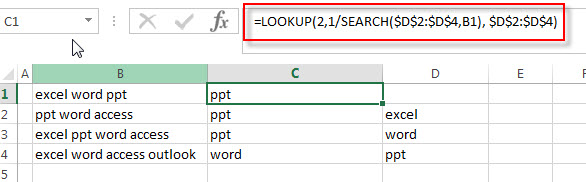# How to get last match that cell contains one of several values in a range

This post explains that how to check a cell that contains one of several values in another range, and then return the last match value.  And we talked that how to test a cell to see that if it contains one of several values in a list in the previous post. And this post will guide you how to get the last match value in the range.

## Get Last Match that cell contains one of several values

If you want to check a cell that if it contains one of several values in a range, you can use the SEARCH function to search find_text in a range inside a text string. Then we can use the LOOKUP function to get the last match values.

For example, you have a list of text strings in the range B1:B4 and you need to check each cell if it contains one of several values in the range D2:D4, if TRUE, then return the last match values from the range D2:D4. You can write down the following formula:

`=LOOKUP(2,1/SEARCH(\$D\$2:\$D\$4,B1), \$D\$2:\$D\$4)`

Let’s see how this formula works:

= SEARCH(\$D\$2:\$D\$4,B1)

This  formula will search each value from the range D2:D4 inside within_text in Cell B1, if it find the matched string, then return the position of the first character in Cell B1, if not, it returns the #VALUE error.  So this formula will return an array result like this:

`{1;7;12}`

=1/SEARCH(\$D\$2:\$D\$4,B1)

The array result returned by the SEARCH function is divided by number 1, then this formula returns another new array result that is composed of the #VALUE! Error and decimal values. If the item is a decimal value, then it indicates that one value found. The array is like this:

`{1;0.142857142857143;0.0833333333333333}`

The returned array result goes into the LOOKUP function as its lookup_vector argument.

=LOOKUP(2,1/SEARCH(\$D\$2:\$D\$4,B1), \$D\$2:\$D\$4)You can see that the value of lookup vector will never larger than 1, and the lookup value is set to 2. So the lookup value is not able to be found. So the LOOKUP function will match the last numeric value in the array result of the lookup_vector, then return the value from the same position in the range D2:D4.

### Related Formulas

• Check If Cell Contains All Values from Range
If you want to check if a cell contains all values in a list, you can use a combination of the SEARCH function, the SUMPRODUCT function, the ISNUMBER function and COUNTA function…
• Get first match that cell contains one of several values in a range
You can use a combination of the INDEX function, the MATCH function, the ISNUMBER function and the SEARCH function to create a new excel array formula to get first match cell contains…
• Check if Cell contains one of many values from range
Assuming that you have a list of text strings in the range B1:B3 and you want to check each text string if it contains one of several values in a range E1:E3. If it contains any of text string in range E1:E3, then it should be return TRUE value, otherwise, it should return FALSE….

### Related Functions

• Excel LOOKUP function
The Excel LOOKUP function will search a value in a vector or array.The syntax of the LOOKUP function is as below:= LOOKUP (lookup_value, lookup_vector, [result_vector])…
• Excel SEARCH function
The Excel SEARCH function returns the number of the starting location of a substring in a text string.The syntax of the SEARCH function is as below:= SEARCH  (find_text, within_text,[start_num])…